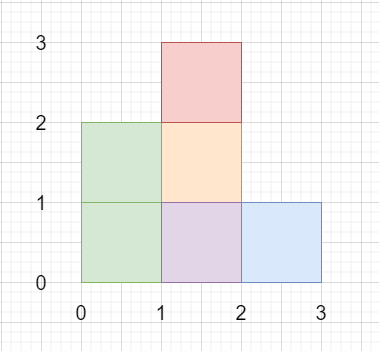# Rectangle Area II in C++

Suppose we have a list of (axis-aligned) rectangles. Here each rectangle[i] = {x1, y1, x2, y2}, where (x1, y1) is the point of the bottom-left corner, and (x2, y2) are the point of the top-right corner of the ith rectangle.

We have to find the total area covered by all rectangles in the plane. The answer may be very , so we can use modulo 10^9 + 7.

So, if the input is likethen the output will be 6.

To solve this, we will follow these steps −

• m = 10^9 + 7

• Define a function add(), this will take a, b,

• return ((a mod m) + (b mod m) mod m)

• Define one function compress this will take 2d matrix v

• Define an array temp

• for initialize i := 0, when i < size of v, update (increase i by 1), do −

• insert v[i, 0] at the end of temp

• insert v[i, 2] at the end of temp

• sort the array temp

• Define one map, ret

• idx := 0

• for initialize i := 0, when i < size of temp, update (increase i by 1), do

• if temp[i] is not member of ret, then −

• ret[temp[i]] := idx

• (increase idx by 1)

• return ret

• From the main method do the following −

• Define an array xv

• insert { 0 } at the end of xv

• for initialize i := 0, when i < size of v, update (increase i by 1), do −

• insert v[i, 0] at the end of xv

• insert v[i, 2] at the end of xv

• sort the array xv

• uniItr = first element of a list with unique elements of xv

• delete uniItr, from xv

• Define one map index

• idx := 0

• for initialize i := 0, when i < size of xv, update (increase i by 1), do −

• index[xv[i]] := i

• Define an array count of size same as index size

• Define one 2D array x

• for initialize i := 0, when i < size of v, update (increase i by 1), do −

• x1 := v[i, 0], y1 := v[i, 1]

• x2 := v[i, 2], y2 := v[i, 3]

• insert { y1, x1, x2, 1 } at the end of x

• insert { y2, x1, x2, -1 } at the end of x

• sort the array x

• ret := 0

• sum := 0, currentY := 0

• for initialize i := 0, when i < size of x, update (increase i by 1), do −

• y := x[i, 0]

• x1 := x[i, 1], x2 := x[i, 2]

• sig := x[i, 3]

• ret := add(ret, (y - currentY) * sum)

• currentY := y

• for initialize i := index[x1], when i < index[x2], update (increase i by 1), do −

• count[i] := count[i] + sig

• sum := 0

• for initialize i := 0, when i < size of count, update (increase i by 1), do −

• if count[i] > 0, then

• sum := sum + (xv[i + 1] - xv[i])

• return ret mod m

Let us see the following implementation to get better understanding −

## Example

Live Demo

#include <bits/stdc++.h>
using namespace std;
typedef long long int lli;
const int m = 1e9 + 7;
class Solution {
public:
return ((a % m) + (b % m) % m);
}
map<int, int> compress(vector<vector<int> >& v){
vector<int> temp;
for (int i = 0; i < v.size(); i++) {
temp.push_back(v[i]);
temp.push_back(v[i]);
}
sort(temp.begin(), temp.end());
map<int, int> ret;
int idx = 0;
for (int i = 0; i < temp.size(); i++) {
if (!ret.count(temp[i])) {
ret[temp[i]] = idx;
idx++;
}
}
return ret;
}
int rectangleArea(vector<vector<int> >& v){
vector<int> xv;
xv.push_back({ 0 });
for (int i = 0; i < v.size(); i++) {
xv.push_back(v[i]);
xv.push_back(v[i]);
}
sort(xv.begin(), xv.end());
vector<int>::iterator uniItr = unique(xv.begin(), xv.end());
xv.erase(uniItr, xv.end());
map<int, int> index;
int idx = 0;
for (int i = 0; i < xv.size(); i++) {
index[xv[i]] = i;
}
vector<int> count(index.size());
vector<vector<int> > x;
int x1, x2, y1, y2;
for (int i = 0; i < v.size(); i++) {
x1 = v[i];
y1 = v[i];
x2 = v[i];
y2 = v[i];
x.push_back({ y1, x1, x2, 1 });
x.push_back({ y2, x1, x2, -1 });
}
sort(x.begin(), x.end());
lli ret = 0;
lli sum = 0, currentY = 0;
for (int i = 0; i < x.size(); i++) {
lli y = x[i];
x1 = x[i];
x2 = x[i];
int sig = x[i];
ret = add(ret, (y - currentY) * sum);
currentY = y;
for (int i = index[x1]; i < index[x2]; i++) {
count[i] += sig;
}
sum = 0;
for (int i = 0; i < count.size(); i++) {
if (count[i] > 0) {
sum += (xv[i + 1] - xv[i]);
}
}
}
return ret % m;
}
};
main(){
Solution ob;
vector<vector<int>> v = {{0,0,2,2},{1,0,2,3},{1,0,3,1}};
cout << (ob.rectangleArea(v));
}

## Input

{{0,0,2,2},{1,0,2,3},{1,0,3,1}}

## Output

6